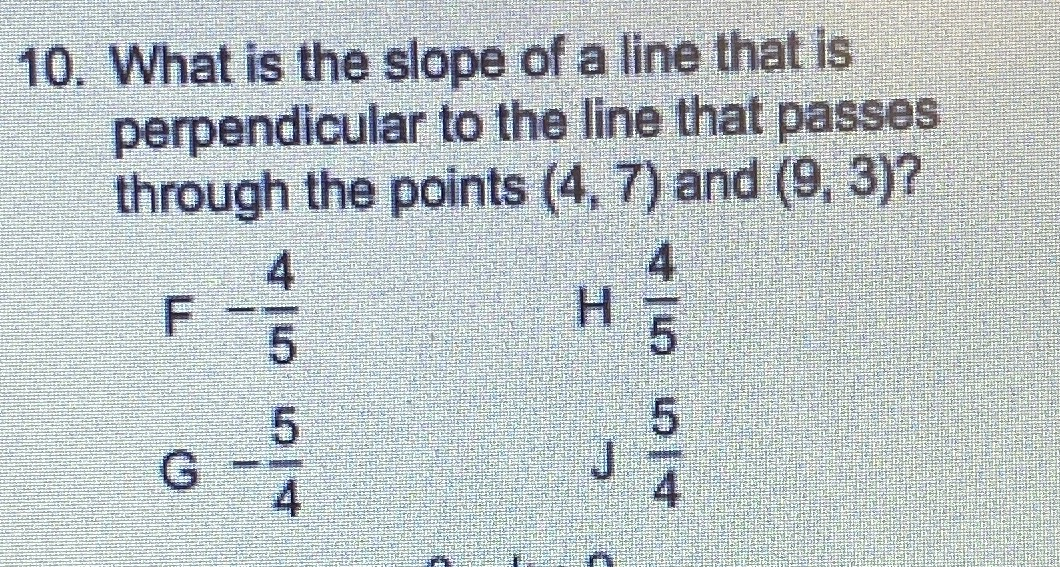### ¿Todavía tienes preguntas de matemáticas?

Pregunte a nuestros tutores expertos
Algebra
Pregunta10. What is the slope of a line that is perpendicular to the line that passes through the points $$( 4,7 )$$ and $$( 9,3 )$$ ?

10. $$Slope = \frac{5}4{}$$